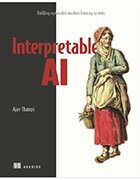# The white-box model approach aims for interpretable AI

## The white-box model approach to machine learning makes AI interpretable since algorithms are easy to understand. Ajay Thampi, author of 'Interpretable AI,' explains this approach.

When building machine learning models or algorithms, developers should adhere to the principle of interpretability so that they and their intended users know exactly how the inputs and inner workings achieve outputs. Interpretable AI is a book written by Ajay Thampi, a machine learning engineer at Meta, and its second chapter explains the white-box model approach to machine learning as well as examples of white-box models.

These models are interpretable, as they feature easy-to-understand algorithms that show how data inputs achieve outputs or target variables. Thampi walks readers through three types of white-box models in this chapter and how they are applied: linear regression, generalized additive models (GAMs) and decision trees.

Given the term regression in machine learning refers to models and algorithms taking data and learning relationships within that data to make predictions, the premise of a linear regression model is that a target prediction variable can be determined as a linear combination of every input variable. Worth noting is that just like the other two types, linear regression deals with supervised data, which is labeled and structured in stark contrast with unsupervised data, a concept that's mostly outside the scope of this book.

While linear regressions are entirely interpretable and explainable, they lack the predictive power that data scientists value in more complex models. Another interpretable model type with far more predictive power is a GAM, which is like a linear regression model, yet it can model nonlinear data and learn nonlinear characteristics. Data structures often have data elements that are not arranged sequentially or linearly, so a GAM would be the solution in such cases.To get a 35% discount
for this book at Manning's
website, use the code
nltechtarget21.

Decision trees can model more complex relationships within data and in addition to being highly interpretable, they also have higher predictive power than linear regression models. The goal of a decision tree is to create a tree-like structure that splits a data set further and further by attributes and features. This is especially useful for the classification of data records, although it's used in regression tasks as well to predict target variables, just like the other model types.

The second chapter further elaborates on these white-box model types and provides a concrete example scenario -- a healthcare center using AI to predict diabetes in patients -- where they would be used. As for the remainder of the book, Thampi offers a wide array of interpretability techniques to help professionals interpret black-box models, which are the opposite of white-box models in that they have algorithms and methodologies that are highly complicated and not easy for observers to understand.